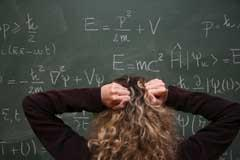Tips for teaching maths skills to our future chemists, by Paul Yates of Keele University

This is a fundamental skill required by chemistry students, principally in order to calculate the values of specified properties. As we will see, this is straightforward, but a number of obstacles do exist before students can feel confident in doing this. For example:

• they may have previously avoided using formal algebraic methods. For example, the equation x + 5 = 12 can be solved by trial and error;
• they may feel uncomfortable using the formal rules.2 We will see that the rules are easily derived, and don't have to be remembered;
• even though the rules are simple, the presence of more complicated functions (such as logarithms or powers) may mask this simplicity;
• they may rely on remembering specific types of problems. There is potentially an infinite number of ways in which an expression may need to be rearranged.4Rearranging equations can be challenging for some

It is also worth noting that by expressions we mean formulae, equations or identities.5 While these do have mathematical differences, from a chemist's point of view they can all be thought of as being any expression with an equals sign (or sense of balance) between two groups of quantities.

### Different methods

Zalman Usiskin5 (director of the University of Chicago Mathematics Project) gives the example:

5x + 3 = 40

and begins to solve it by adding -3 to each side to give

5x = 37

Usiskin suggests that the number of steps required to get to this point depends on the level of student and preference of the teacher. This is essentially using the balance model1 found in many textbooks, in which both sides of an equation are treated identically. This is a powerful method that has the further advantage of being easy to remember.

Alternatively a rules-based approach can be used. This is based on the fact that when a quantity is moved from one side of an equation to the other:

• positive terms become negative and vice versa;
• numerators become denominators and vice versa.

My own experience suggests that many students feel more comfortable using a rule-based approach to rearrangement, even though the balance method is more logical and possibly easier to apply in more complicated situations. The two methods, however, are compatible. In both cases it is important to remember that the rules of precedence apply.

### Example 1: The phase rule

This is possibly the simplest relationship found in physical chemistry because it relates the number of degrees of freedom F, the number of components C and the number of phases P by the linear equation:

F = C - P + 2

Let's suppose that we want to rearrange this to make C the subject. If we add P to both sides of this equation and subtract two we have

F + P - 2 = C - P + 2 + P - 2

which simplifies to

F + P - 2 = C

and would more conventionally be written as

C = F + P - 2

A rules-based approach would move -P to the left-hand side of the original equation to become +P, and move +2 to the left-hand side of the equation to become -2, giving the same result.

The frequency ν and wavelength λ of electromagnetic radiation are related to its velocity c by the equation:

c = νλ

If we want to obtain an expression for λ we can divide both sides of the equation by ν to give

c / ννλ ν

which simplifies to

c / ν = λ

or written more conventionally as

λ c / ν

In terms of a rules-based approach we would say that v moves from the top on the right-hand side of the equation to the bottom on the left.

This relationship also leads to a further example of rearrangement. The wavenumber ṽ is defined by the relationship:

ṽ = 1 / λ

Since λ= c / v we can take the reciprocal of both sides of this equation to give

1 / λ= v c

and consequently

ṽ = c

The rules used in these two examples are both easily deduced from the balance approach, and it would seem to be sensible to introduce students to the rules in this way, if required.

### Example 3: The Nernst equation

This example contains a difference, product and quotient, as well as a logarithmic term. It gives the electromotive force E in terms of that of a cell under standard condition Eo, the gas constant R, absolute temperature T, numerical amount of charge transferred n, Faraday constant F and the reaction quotient Q as:

E = Eo - RT/nF lnQ

This will be rearranged to make lnQ the subject. The first step in doing this is to subtract Eo from each side to give

E - Eo = Eo - RT/nF lnQ - Eo

which simplifies to

E - E = - RT/nF lnQ

Both sides can now be multiplied by -1 to give

-(EEo) = - (-RT/n F lnQ)

or

Eo - E = RT/nF lnQ

Both sides of this equation are now multiplied by n F/RT:

nF/RT (Eo - E) = nF/RT × nF/RT (lnQ)

or

nF/RT(Eo - E) = lnQ

which would conventionally be written as

lnQ = n F/RT (EoE)

Note that the fact that a logarithmic term is involved in this equation is irrelevant for the manipulations. This could, however, be a source of complication for students who have failed to adopt a strategic approach.